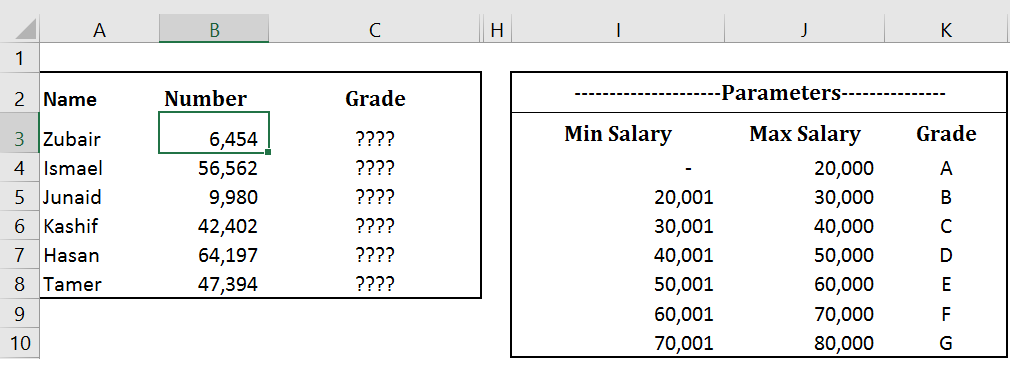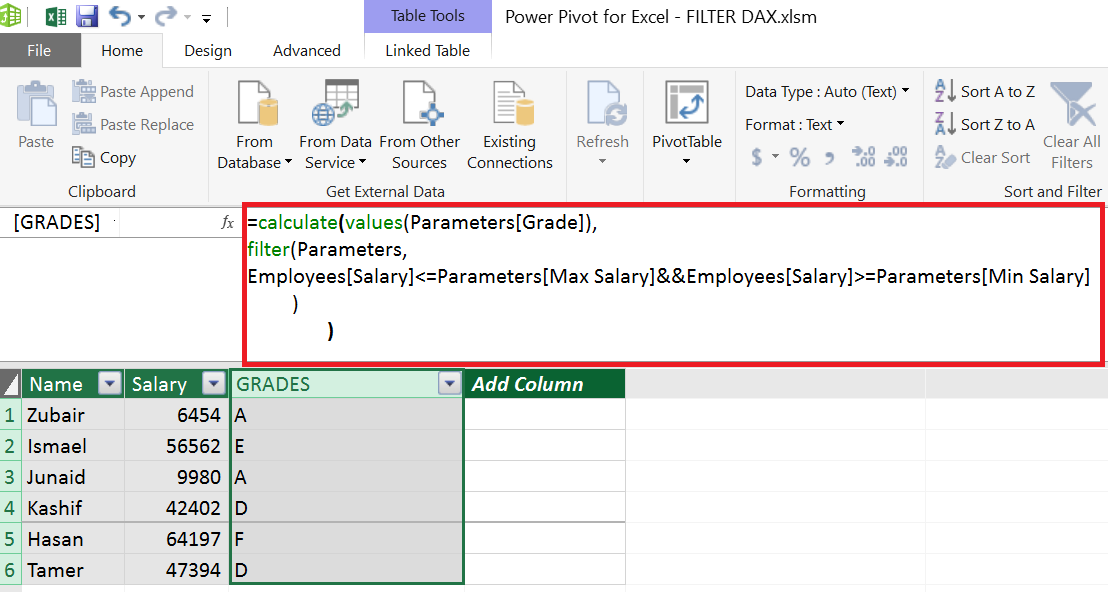# FILTER CONNECTS UNRELATED TABLES

One important feature of FILTER function in DAX is that it can establish a relationship between two tables when no direct relationship (common Field) exists between them.

In my post on Array Formulas, I used following two tables and showed how Index Match Array Formula is used to look up Employee Grade. Obviously normal VLookup or Index Match cannot be performed due to absence of common field between the tables.In Power Pivot we do not have the luxury of array formulas but we have the powerful DAX’s FILTER function which can iterate through the LookUp Table matching multiple set of criterion and returning the rows where criterion are met.

Since we need the  grades in  Employee’s Table, we will create a calculated column here. The filter function that will return the relevant row from the Parameter Table is as follows

FILTER (Parameters ,
Employees[Salary]<=Parameters[Max Salary]  &&  Employees[Salary]>=Parameters[Min Salary]
)

Filter returns a Table expression, so it cannot be used directly inside the calculated column. Since we are sure that only row from Parameter Table is returned by filter table (each employee can have 1 Grade only), we can use combination of CALCULATE and VALUES to get the GRADE from the filtered table

Note: VALUES(Table(Column)) returns a scalar value if the Table has only 1 row

So our final DAX formula looks like thisTo conclude, In Normal Excel we use Vlookup or Index-Match to lookup values from  related tables. In Power Pivot we use DAX functions “RELATED” and “RELATEDTABLE” to do the same.

When there is no direct relationship (common field) between the tables we can use ARRAY formulas in Excel to lookup data. In Power Pivot, FILTER function assisted by CALCULATE and VALUES can do the same job elegantly.